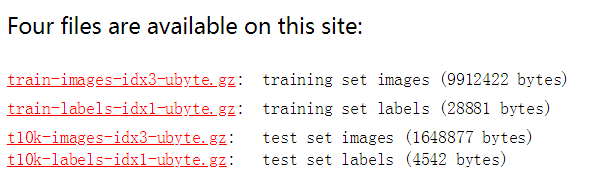• mnist数据集包括训练图像数据，训练图像的标签，测试图像数据，测试图像标签
• MNIST数据集是一个入门级的计算机视觉数据集，数据集中都是美国中学生手写的数字，其中训练集包括6万张图片，测试集包括1万张图片，并且数字已经进行过预处理和格式化。
• mnist数据集用于训练手写数字识别，数据集中有 60000个训练样本，10000个测试样本。注意图片和label是分开的，而且需要用代码进行解析（diamante很简单，搜索下即可）
• mnist数据集是由深度学习大神 LeCun等人制作完成的数据集，mnist数据集也常认为是深度学习的“ Hello World！”。 官网：http://yann.lecun.com/exdb/mnist/ mnist数据集由6万张训练数据和1万张测试数据组成。 ...
mnist数据集是由深度学习大神 LeCun等人制作完成的数据集，mnist数据集也常认为是深度学习的“ Hello World！”。

官网：http://yann.lecun.com/exdb/mnist/

mnist数据集由6万张训练数据和1万张测试数据组成。官网提供下载，但由于是国外的服务器，下载速度很慢。这里提供百度网盘下载地址：

链接：https://pan.baidu.com/s/17KUWe8JdQBHsAg3B4m5SNg
提取码：wyxn


展开全文深度学习 MNIST
• ## 详解 MNIST数据集

万次阅读 多人点赞 2017-07-17 20:41:35
MNIST 数据集已经是一个被”嚼烂”了的数据集, 很多教程都会对它”下手”, 几乎成为一个 “典范”. 不过有些人可能对它还不是很了解, 下面来介绍一下.MNIST 数据集可在 http://yann.lecun.com/exdb/mnist/ 获取, 它...
MNIST 数据集已经是一个被”嚼烂”了的数据集, 很多教程都会对它”下手”, 几乎成为一个 “典范”. 不过有些人可能对它还不是很了解, 下面来介绍一下.

MNIST 数据集可在 http://yann.lecun.com/exdb/mnist/ 获取, 它包含了四个部分:

Training set images: train-images-idx3-ubyte.gz (9.9 MB, 解压后 47 MB, 包含 60,000 个样本)
Training set labels: train-labels-idx1-ubyte.gz (29 KB, 解压后 60 KB, 包含 60,000 个标签)
Test set images: t10k-images-idx3-ubyte.gz (1.6 MB, 解压后 7.8 MB, 包含 10,000 个样本)
Test set labels: t10k-labels-idx1-ubyte.gz (5KB, 解压后 10 KB, 包含 10,000 个标签)
MNIST 数据集来自美国国家标准与技术研究所, National Institute of Standards and Technology (NIST). 训练集 (training set) 由来自 250 个不同人手写的数字构成, 其中 50% 是高中学生, 50% 来自人口普查局 (the Census Bureau) 的工作人员. 测试集(test set) 也是同样比例的手写数字数据.

不妨新建一个文件夹 – mnist, 将数据集下载到 mnist 以后, 解压即可:图片是以字节的形式进行存储, 我们需要把它们读取到 NumPy array 中, 以便训练和测试算法.

import os
import struct
import numpy as np

"""Load MNIST data from path"""
labels_path = os.path.join(path,
'%s-labels-idx1-ubyte'
% kind)
images_path = os.path.join(path,
'%s-images-idx3-ubyte'
% kind)
with open(labels_path, 'rb') as lbpath:
magic, n = struct.unpack('>II',
labels = np.fromfile(lbpath,
dtype=np.uint8)

with open(images_path, 'rb') as imgpath:
magic, num, rows, cols = struct.unpack('>IIII',
images = np.fromfile(imgpath,
dtype=np.uint8).reshape(len(labels), 784)

return images, labels

load_mnist 函数返回两个数组, 第一个是一个 n x m 维的 NumPy array(images), 这里的 n 是样本数(行数), m 是特征数(列数). 训练数据集包含 60,000 个样本, 测试数据集包含 10,000 样本. 在 MNIST 数据集中的每张图片由 28 x 28 个像素点构成, 每个像素点用一个灰度值表示. 在这里, 我们将 28 x 28 的像素展开为一个一维的行向量, 这些行向量就是图片数组里的行(每行 784 个值, 或者说每行就是代表了一张图片). load_mnist 函数返回的第二个数组(labels) 包含了相应的目标变量, 也就是手写数字的类标签(整数 0-9).

第一次见的话, 可能会觉得我们读取图片的方式有点奇怪:

magic, n = struct.unpack('>II', lbpath.read(8))
labels = np.fromfile(lbpath, dtype=np.uint8)

为了理解这两行代码, 我们先来看一下 MNIST 网站上对数据集的介绍:

TRAINING SET LABEL FILE (train-labels-idx1-ubyte):

[offset] [type]          [value]          [description]
0000     32 bit integer  0x00000801(2049) magic number (MSB first)
0004     32 bit integer  60000            number of items
0008     unsigned byte   ??               label
0009     unsigned byte   ??               label
........
xxxx     unsigned byte   ??               label
The labels values are 0 to 9.

通过使用上面两行代码, 我们首先读入 magic number, 它是一个文件协议的描述, 也是在我们调用 fromfile 方法将字节读入 NumPy array 之前在文件缓冲中的 item 数(n). 作为参数值传入 struct.unpack 的 >II 有两个部分:

>: 这是指大端(用来定义字节是如何存储的); 如果你还不知道什么是大端和小端, Endianness 是一个非常好的解释. (关于大小端, 更多内容可见<<深入理解计算机系统 – 2.1 节信息存储>>)
I: 这是指一个无符号整数.
通过执行下面的代码, 我们将会从刚刚解压 MNIST 数据集后的 mnist 目录下加载 60,000 个训练样本和 10,000 个测试样本.

为了了解 MNIST 中的图片看起来到底是个啥, 让我们来对它们进行可视化处理. 从 feature  matrix 中将 784-像素值 的向量 reshape 为之前的 28*28 的形状, 然后通过 matplotlib 的 imshow 函数进行绘制:

import matplotlib.pyplot as plt

fig, ax = plt.subplots(
nrows=2,
ncols=5,
sharex=True,
sharey=True, )

ax = ax.flatten()
for i in range(10):
img = X_train[y_train == i].reshape(28, 28)
ax[i].imshow(img, cmap='Greys', interpolation='nearest')

ax.set_xticks([])
ax.set_yticks([])
plt.tight_layout()
plt.show()

我们现在应该可以看到一个 2*5 的图片, 里面分别是 0-9 单个数字的图片.此外, 我们还可以绘制某一数字的多个样本图片, 来看一下这些手写样本到底有多不同:

fig, ax = plt.subplots(
nrows=5,
ncols=5,
sharex=True,
sharey=True, )

ax = ax.flatten()
for i in range(25):
img = X_train[y_train == 7][i].reshape(28, 28)
ax[i].imshow(img, cmap='Greys', interpolation='nearest')

ax.set_xticks([])
ax.set_yticks([])
plt.tight_layout()
plt.show()

执行上面的代码后, 我们应该看到数字 7 的 25 个不同形态:另外, 我们也可以选择将 MNIST 图片数据和标签保存为 CSV 文件, 这样就可以在不支持特殊的字节格式的程序中打开数据集. 但是, 有一点要说明, CSV 的文件格式将会占用更多的磁盘空间, 如下所示:

train_img.csv: 109.5 MB
train_labels.csv: 120 KB
test_img.csv: 18.3 MB
test_labels: 20 KB
如果我们打算保存这些 CSV 文件, 在将 MNIST 数据集加载入 NumPy array 以后, 我们应该执行下列代码:

np.savetxt('train_img.csv', X_train,
fmt='%i', delimiter=',')
np.savetxt('train_labels.csv', y_train,
fmt='%i', delimiter=',')
np.savetxt('test_img.csv', X_test,
fmt='%i', delimiter=',')
np.savetxt('test_labels.csv', y_test,
fmt='%i', delimiter=',')

一旦将数据集保存为 CSV 文件, 我们也可以用 NumPy 的 genfromtxt 函数重新将它们加载入程序中:

X_train = np.genfromtxt('train_img.csv',
dtype=int, delimiter=',')
y_train = np.genfromtxt('train_labels.csv',
dtype=int, delimiter=',')
X_test = np.genfromtxt('test_img.csv',
dtype=int, delimiter=',')
y_test = np.genfromtxt('test_labels.csv',
dtype=int, delimiter=',')

不过, 从 CSV 文件中加载 MNIST 数据将会显著发给更长的时间, 因此如果可能的话, 还是建议你维持数据集原有的字节格式.

参考:
- Book , Python Machine Learning.
展开全文python 机器学习 深度学习
• 一、MNIST数据集分类简单版本import tensorflow as tffrom tensorflow.examples.tutorials.mnist import input_data#载入数据集mnist = input_data.read_data_sets("MNIST_data",one_hot=True)#每个批次的大小batch_...
一、MNIST数据集分类简单版本import tensorflow as tffrom tensorflow.examples.tutorials.mnist import input_data#载入数据集mnist = input_data.read_data_sets("MNIST_data",one_hot=True)#每个批次的大小batch_size = 100#计算一共有多少个批次n_batch = mnist.train.num_examples // batch_size#定义两个placeholderx = tf.placeholder(tf.float32,[None,784])y = tf.placeholder(tf.float32,[None,10])#创建一个简单的神经网络W = tf.Variable(tf.zeros([784,10]))b = tf.Variable(tf.zeros())prediction = tf.nn.softmax(tf.matmul(x,W)+b)#二次代价函数loss = tf.reduce_mean(tf.square(y-prediction))#使用梯度下降法train_step = tf.train.GradientDescentOptimizer(0.2).minimize(loss)#初始化变量init = tf.global_variables_initializer()#结果存放在一个布尔型列表中correct_prediction = tf.equal(tf.argmax(y,1),tf.argmax(prediction,1))#argmax返回一维张量中最大的值所在的位置#求准确率accuracy = tf.reduce_mean(tf.cast(correct_prediction,tf.float32))with tf.Session() as sess:sess.run(init)for epoch in range(21):for batch in range(n_batch):batch_xs,batch_ys =  mnist.train.next_batch(batch_size)sess.run(train_step,feed_dict={x:batch_xs,y:batch_ys})acc = sess.run(accuracy,feed_dict={x:mnist.test.images,y:mnist.test.labels})print("Iter " + str(epoch) + ",Testing Accuracy " + str(acc))展开全文• 1.一开始采用官网上利用input_data来...2.后面采用 keras加载mnist数据集，又会报出如下错误 URL fetch fail:http://googelsourc…………………………………… 解决办法：先将mnist数据集下载到本地，放到自己想...
注意：使用keras模块加载mnist数据集时，可以不用修改代码，直接将mnist数据集放到相应的路径下面。
windows: C:\Users\mac\.keras\datasets

1.一开始采用官网上利用input_data来加载本地数据集的方法，但会报出下面的错误
No module named 'tensorflow.examples.tutorials'

并且官网上input_data.py又下载不下来
解决方法：修改mnist.py（使用 ctrl + b，打开mnist.py），利用本地下载好的mnist数据集，直接将mnist.py里路径path改成本地mnist数据集的路径
下附代码：
main.py
from __future__ import absolute_import, division, print_function, unicode_literals
import tensorflow as tf

mnist = tf.keras.datasets.mnist

(x_train, y_train), (x_test, y_test) = mnist.load_data()
x_train, x_test = x_train / 255.0, x_test / 255.0

model = tf.keras.models.Sequential([
tf.keras.layers.Flatten(input_shape=(28, 28)),
tf.keras.layers.Dense(128, activation='relu'),
tf.keras.layers.Dropout(0.2),
tf.keras.layers.Dense(10, activation='softmax')
])

loss='sparse_categorical_crossentropy',
metrics=['accuracy'])

model.fit(x_train, y_train, epochs=5)

model.evaluate(x_test,  y_test, verbose=2)


mnist.py

"""MNIST handwritten digits dataset.
"""
from __future__ import absolute_import
from __future__ import division
from __future__ import print_function

import numpy as np

from tensorflow.python.keras.utils.data_utils import get_file
from tensorflow.python.util.tf_export import keras_export

Arguments:
path: path where to cache the dataset locally
(relative to ~/.keras/datasets).

Returns:
Tuple of Numpy arrays: (x_train, y_train), (x_test, y_test).

Yann LeCun and Corinna Cortes hold the copyright of MNIST dataset,
which is a derivative work from original NIST datasets.
MNIST dataset is made available under the terms of the
"""

path = "./mnist.npz"
x_train, y_train = f['x_train'], f['y_train']
x_test, y_test = f['x_test'], f['y_test']

return (x_train, y_train), (x_test, y_test)



展开全文数据集
• 本节基于回归学习对 MNIST 数据集进行处理，但将添加一些 TensorBoard 总结以便更好地理解 MNIST 数据集。(来源https://www.tensorflow.org/get_started/mnist/beginners)大部分人已经对 MNIST 数据集很熟悉了，它是...
• 上传一个mnist合集的数据文件，里面包括 1 tensorflow里使用的mnist数据集， 2 keras使用的mnist数据集mnist.npz 3 手写数字识别demo里需要的数据集mnist.pkl.gz
• 1. MNIST数据集加载与查看 1.1 内置数据集介绍 dir(tf.keras.datasets) 1.2 MNIST数据集加载 mnist = tf.keras.datasets.mnist mnist.load_data() 1.3 数据集查看 x_train.shape, y_train.shape import ...
• fashion-MNIST数据集的加载方法与MNIST数据集类似。2017年8月27日，Fashion-MNIST图片库在GitHub上开源，MNIST的时代宣告终结。fashion_mnist数据集的训练难度比MNIST大得多，但是也有意义去学习。
• ## 模仿mnist数据集制作自己的数据集

万次阅读 多人点赞 2017-08-05 20:18:28
模仿mnist数据集制作自己的数据集 最近看深度学习，用TensorFlow跑教程里的模型，发现教程里大多都是用mnist和cifar这两个数据集来演示的。想测试自己的数据集看看初步效果，于是就想套用现有的模型，将自己的数据集...制作
• '''将二进制格式的MNIST数据集转成.jpg图片格式并保存，图片标签包含在图片名中''' import numpy as np import cv2 import os import struct def save_mnist_to_jpg(mnist_image_file, mnist_label_file, save_dir)...struct python...